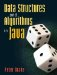# A.8. Operators

Operators are built-in functions for manipulating (usually primitive) values. In Java, we can define new methods, but we cannot define new operators.

Arithmetic Operators

Arithmetic operators are summarized in Figure A-18.

Figure A-18. Arithmetic operators.

(This item is displayed on page 534 in the print version)

Operator

Description

Notes

+

chars can be added with Strings, this is the concatenation operator

-

subtraction

can be applied to a single value (for example, -x) chars can be subtracted

*

multiplication

/

division

%

remainder

behaves like modulo with positive numbers

These generally behave as one would expect, but we must remember the following:

• It is legal to do arithmetic with chars. For example 'c' - 'a' is 2. This is particularly useful when converting between alphabetic and numeric indices. If we are given a char letter, then letter - 'a' is 0 if letter is 'a', 1 if letter is 'b', and so on.
• Adding two Strings together concatenates them into one longer String.
• When working with ints, division discards any remainder.
• The % operator, sometimes called modulo, returns the remainder of a division. For example, 27 % 10 is 7. (It is not exactly the same as the mod operator as used by mathematicians. For example, -2 % 10 is 2, but 2 mod 10 is 8.)
• There is an operator precedence hierarchy specifying that multiplication happens before addition and so on. Since Java has so many operators, the complete hierarchy has 14 levels. Rather than trying to memorize it and expecting anyone reading our code to do the same, we use parentheses to ensure that operations happen in the correct order.

Assignment Operators

Assignment operators are used to set or change the value of a variable. They are summarized in Figure A-19.

Figure A-19. Assignment operators.

(This item is displayed on page 535 in the print version)

Operator

Description

Notes

=

assignment

returns a value

++

increment

returns a value, but the value should not be used

--

decrement

returns a value, but the value should not be used

+=

increment by

x += y is equivalent to x = x + y

-=

decrement by

x -= y is equivalent to x = x - y

*=

multiply by

x *= y is equivalent to x = x * y

/=

divide by

x /= y is equivalent to x = x / y

%=

remainder by

x %= y is equivalent to x = x % y

Things to watch out for:

• The = operator returns the value of its right-hand operand. One consequence is that it is legal to assign the same value to several variables like this:

```x = (y = (z = 3));
```

It is okay to omit the parentheses in this case:

```x = y = z = 3;
```

A second consequence is that if we accidentally type

```if (love = true) { ... }
```

when we mean to type

```if (love == true) { ... }
```

then the test will always be passed, because love = true is an assignment statement that returns true. We can avoid this issue by using the equivalent code:

```if (love) { ... }
```
• Suppose x is 2 and we evaluate the statement:

```y = x++;
```

Now x is 3, but what is y? There is an answer, but it's not immediately obvious whether it's 2 or 3. Worse, the answer is different for:

```y = ++x;
```

Rather than ask anyone reading our programs to perform these mental gymnastics, we'll just avoid using the return value of a ++ or -- expression.

Comparison Operators

These operators, used to compare values, are summarized in Figure A-20.

Figure A-20. Comparison operators.

(This item is displayed on page 536 in the print version)

Operator

Description

Notes

==

equality

use only with primitive values

!=

inequality

use only with primitive values

<

less than

<=

less than or equal to

>

greater than

>=

greater than or equal to

The only thing to watch out for here is that == and != should be used only to compare primitive values. If they are used to compare (for example) Strings, strange things can happen. This is explained in much more detail in Chapter 2.

Logical Operators

Figure A-21 shows some operators for manipulating boolean values.

Figure A-21. Logical operators.

(This item is displayed on page 536 in the print version)

Operator

Description

Notes

&&

and

short-circuited

||

or

short-circuited

!

not

The && and || operators are short-circuited, meaning that Java stops as soon as it knows the answer. In addition to saving computation time, this is particularly useful for expressions such as:

```(bears.length > 10) && (bears == "hungry")
```

If the left side evaluates to false, the entire expression must evaluate to false, so Java does not bother evaluating the right side. Since the right side is not evaluated, it does not cause a problem if there is no such element as bears.

Perversely, if you accidentally type & instead of && (or | instead of ||), the Java compiler won't complainbut you'll get a non-short-circuited version.

Java has a few other operators, but they are fairly obscure. The only other ones we will use in this book are the bitwise operators discussed in Section 12.1.

Exercises

 A.17 What is the value of 3 / 4? How about 3.0 / 4.0? A.18 What is the value of 123 % 20? A.19 Which assignment operators (Figure A-19) can be used to initialize a variable? A.20 Change line 15 of Figure A-11 to read: ```if (comparison = '<') { ```   Why is this an error? Does the compiler catch it? A.21 Write a program which contains the erroneous expression args[-1] == args but still compiles and runs. (Hint: Use a short-circuited logical operator.)Data Structures and Algorithms in Java
ISBN: 0131469142
EAN: 2147483647
Year: 2004
Pages: 216
Authors: Peter Drake